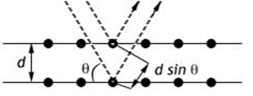Plasma Laboratory - Weizmann Institute of Science

## X-ray spectroscopy

When x-rays are diffracted from a crystal surface, each plane of crystal atoms reflects the wave. The separately reflected waves will remain in phase if their respective path lengths differ by an integer multiple of the wavelength. The paths of two such waves are shown in Fig. 1.Fig. 1 - Bragg diffraction

Bragg’s diffraction law dictates when the waves remain in phase:

2d sin θ = nλ,

where n , θ, d, and λ are the (integer) diffraction order, diffraction angle, lattice spacing, and the wavelength, respectively. When the waves are in phase upon reaching the detection plane, maximum intensity is obtained.

• X-ray spectroscopy

^ Top
Modified on: 2010-05-23